Published: 08 December 2016

# Stability enhancement method and experiment of orchard vehicle control

Xin Wang1
Yuding Bai2
Yameng Jiang3
Peng Qiu4
Nannan Du5
1, 2, 3, 4, 5Beijing Key Laboratory of Optimized Design for Modern Agricultural Equipment, College of Engineering, China Agricultural University, Beijing 100083, China
Corresponding Author:
Xin Wang
Views 28

#### Abstract

Study on orchard working vehicle rollover and tipping prediction is important to maintain vehicle stability control in complicated operation conditions of orchard. Existing rollover and tipping prediction models for vehicles can not directly apply to orchard working vehicle, which structure and loading are changing under operation. So it is necessary to move ahead study on orchard vehicle bodywork posture prediction and rollover and tipping prediction by theoretical analysis, mathematical modelling, real vehicle test and other methods. In this paper, firstly, we establish orchard working vehicle dynamic model, analyses variation of key parameters during vehicle instability state, and look for characteristic parameters of vehicle instability. Secondly, active safety control algorithm which based on posture detection of vehicle body is researched. Finally, control model is verified and optimized by scaled test.

## 1. Introduction

Orchard vehicle, as a typical agricultural and versatile machine, has a lot of different tasks to carry out, such as seeding, spraying, fertilization, pruning and harvesting, etc. As the orchard vehicle often worked on sloping or uneven ground, and the center of mass is raised by lift platform, orchard vehicle rollovers cause over 45 % of fatalities in agricultural accidents each year . The stability loss on rough ground is mainly because wheels follow bumps and hollows of rough ground and cause the instable of the vehicle . So, it is very useful to design a control method which is able to keep orchard vehicle stable in various situations.

Nowadays, rollover protective structure (ROPS) is very popular in protecting the driver from rollover accidents [3-5]. Thambiratnam  researched on the performance of bulldozer rollover protective structure, and did a lot of work to solve the contradictory between ROPS bearing requirements and energy absorption requirements. Clark  extracted ROPS energy absorbing components for dynamic simulation analysis; he installed a thin cone tube as the energy absorption component on every side of ROPS to improve the energy absorption capacity. In order to study the behaviour of the ROPS mechanism in dynamic and static loading, Clark  also use finite element technology to simulate the nonlinear response of agricultural vehicles ROPS which is under the lateral concentrated static load.

But orchard vehicle has to be move freely between canopies of trees; it is impractical to be equipped with a ROPS. To provide necessary protection to drivers working in such a confined and complex environment, researchers should develop an active safety control method [9-12].

The goal of this paper is to design an active safety control method for typical orchard vehicle, which can suitable for its rough and changeable working environment. The experiment of control system, sloped surface and obstacles are carried out and the feasibility of control algorithmic verified.

## 2. Vehicle model

Ignoring the influence of non-sprung mass and lateral wind, considering the nonlinear factors such as front steering angle and the coupling of vertical movement and lateral movement when braking [13-16], 5 degree of freedom orchard vehicle rollover dynamics model has been set up which is shown in Fig. 1.

The dynamics differential equations ca n be got from Fig. 1 as following:

Longitudinal motion equation:

1
$m{a}_{x}=-\left({f}_{x1}+{f}_{x2}\right)\mathrm{cos}\delta -\left({f}_{y1}+{f}_{y2}\right)\mathrm{sin}\delta -\left({f}_{x3}+{f}_{x4}\right).$

Lateral movement equation:

2
$m{a}_{y}-msh{\phi }_{y}=\left({f}_{y1}+{f}_{y2}\right)\mathrm{cos}\delta -\left({f}_{x1}+{f}_{x2}\right)\mathrm{sin}\delta -\left({f}_{y3}+{f}_{y4}\right).$

Yaw motion equation:

3
${I}_{z}\stackrel{˙}{r}=\left[\left({f}_{y1}+{f}_{y2}\right)\mathrm{cos}\delta -\left({f}_{x1}+{f}_{x2}\right)\mathrm{sin}\delta -\left({f}_{y3}+{f}_{y4}\right)b$
$\mathrm{}\mathrm{}\mathrm{}\mathrm{}\mathrm{}+\left[\left({f}_{x2}-{f}_{x1}\right)\mathrm{cos}\delta +\left({f}_{y2}-{f}_{y1}\right)\mathrm{sin}\delta +{f}_{x4}-{f}_{x3}\right]{l}_{\omega }}{2}.$

Roll motion equation:

4
$\left({I}_{x}+{m}_{s}{h}^{2}\right){\stackrel{¨}{\phi }}_{y}-{m}_{s}h{a}_{y}={m}_{s}gh{\phi }_{y}-{c}_{\phi y}{\stackrel{˙}{\phi }}_{y}-{k}_{\phi y}{\phi }_{y}.$

Pitch motion equation:

5
$\left({I}_{y}+{m}_{s}{h}^{2}\right){\stackrel{¨}{\phi }}_{x}-{m}_{s}h{a}_{x}={m}_{s}gh{\phi }_{x}-{c}_{\phi x}{\stackrel{˙}{\phi }}_{x}-{k}_{\phi x}{\phi }_{x},$

where, $m$ is mass of orchard vehicle, ${m}_{s}$ is vehicle sprung mass, ${a}_{x}$, ${a}_{y}$ are vehicle acceleration along the direction of the $x$ and $y$, $r$ is yaw velocity, ${\phi }_{y}$ is roll angle, ${\phi }_{x}$ is pitch angle, $a$, $b$ are respectively distance from center of mass to epipodium and back shaft, ${I}_{x}$, ${I}_{z}$ are vehicle inertia around $X$ axis and $Z$ axis, $\delta$ is steering angle of front wheel; $h$, ${h}_{cm}$ are vehicle roll length and height of the center of gravity, ${k}_{\phi y}$ is suspension equivalent roll stiffness, ${k}_{\phi x}$ is suspension equivalent pitch stiffness, ${c}_{\phi y}$ is suspension equivalent roll damping, ${c}_{\phi x}$ is suspension equivalent pitch damping, ${l}_{w}$ is vehicle width, ${f}_{xi}$, ${f}_{yi}$ ($i=$1,…, 4) is braking force and lateral force for each tire.

Fig. 1Five-DOF model of orchard vehicle rollover and tip-over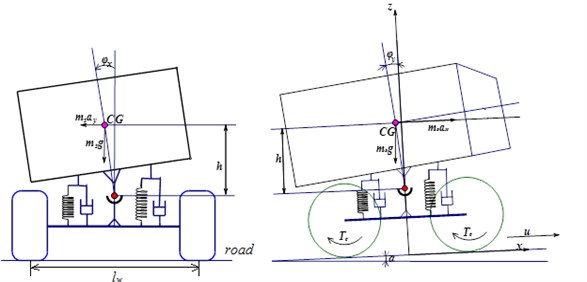## 3. Orchard vehicle bodywork posture control algorithm

Three axis accelerometer and gyro scope module of active safety control system (will describe in following section), which is installed in the orchard vehicle centroid, is used to obtain current $X$ axis angle ${\theta }_{x}$ and angular velocity ${\theta }_{x}^{\text{'}}$, $Y$ axis angle ${\theta }_{y}$ and angular velocity ${\theta }_{y}^{\text{'}}$.

By processing and analysing points sampling data, orchard vehicle ratio coefficient matrix of $X$ axis and $Y$ axis, ${K}_{x}=\left[{k}_{x1},{k}_{x2}\right]$ and ${K}_{y}=\left[{k}_{y1},{k}_{y2}\right]$, can be determined. ${k}_{x1}$ is restoring force coefficient of $X$ axis direction and ${k}_{x2}$ is damping coefficient of $X$ axis direction. ${k}_{y1}$ is restoring force coefficient of the $Y$ axis direction and ${k}_{y2}$ is damping coefficient of $Y$ axis direction.

After that, obtain $\left(k-1\right)$-point estimating value matrixes of angle and angular velocity of $X$ and $Y$ axes ${X}_{\left(k-1\right)x}=\left[{\theta }_{\left(k-1\right)x},{\theta }_{\left(k-1\right)x}^{\text{'}}\right]$ and ${X}_{\left(k-1\right)y}=\left[{\theta }_{\left(k-1\right)y},{\theta }_{\left(k-1\right)y}^{\text{'}}\right]$ with $\mathrm{k}$points sampling data. ${\theta }_{\left(k-1\right)x}$ is estimated value of angle $X$ axis at sample time $k-1$, ${\theta }_{\left(k-1\right)y}^{\text{'}}$ is estimated value of the angular velocity of $X$ axis of $k-1$ points, ${\theta }_{\left(k-1\right)y}$ is $\left(k-1\right)$-point estimated value of angle $Y$ axis, ${\theta }_{\left(k-1\right)y}^{\text{'}}$ is $\left(k-1\right)$-point estimated value of the angular velocity of $Y$ axis.

Then, using apriori estimate covariance matrix on $X$ axis and $Y$ axis ${Q}_{\left(k-1\right)x}$ and ${Q}_{\left(k-1\right)y}$, and measurement variance matrix of orchard vehicle posture control system ${P}_{zx}$, ${P}_{zy}$ to calculate $k$-point estimation gain matrix ${k}_{gx}$ and ${k}_{gy}$:

6
${k}_{gkx}=\left[{k}_{g\theta kx},{k}_{g{\theta }^{\text{'}}kx}\right]={I}^{T}\frac{I×{Q}_{\left(k-1\right)x}}{I×\left({Q}_{\left(k-1\right)x}+{P}_{zx}\right)},$
7
${k}_{gky}=\left[{k}_{g\theta ky},{k}_{g{\theta }^{\text{'}}ky}\right]={I}^{T}\frac{I×{Q}_{\left(k-1\right)y}}{I×\left({Q}_{\left(k-1\right)y}+{P}_{zy}\right)}\mathrm{}\mathrm{},$

where, ${k}_{g\theta kx}$ is $k$-point estimates of gain of $X$ axis angle, ${k}_{g\theta \text{'}kx}$ is $k$-point estimates of gain of $X$ axis angle angular velocity, $I=\left[1,0\right]$, ${k}_{g\theta ky}$, ${k}_{g\theta kx}$ is $k$-point estimates of gain of $Y$ axis angle, ${k}_{g\theta \text{'}ky}$ is $k$-point estimates of gain of $Y$ axis angle angular velocity.

Obtain measurement results matrix ${Z}_{kx}=\left[{Z}_{k\theta x},{Z}_{k\theta \text{'}x}\right]\text{,}$${Z}_{ky}=\left[{Z}_{k\theta y},{Z}_{k\theta \text{'}y}\right]$ with the measurement results of orchard vehicle posture control system. ${Z}_{k\theta x}$ is $k$-point dip direction of $X$ axis, ${Z}_{k\theta \text{'}x}$ is $k$-point angular velocity of $X$ axis, ${Z}_{k\theta y}$ is $k$-point dip direction of $Y$ axis, ${Z}_{k\theta \text{'}y}$ is $k$-point angular velocity of $Y$ axis.

Solve $\mathrm{k}$-point estimated value matrix of angle and angular velocity ${X}_{kx}$ and ${X}_{ky}$:

8
${X}_{kx}=\left[{\theta }_{kx},{\theta }_{kx}^{\text{'}}\right]={X}_{\left(k-1\right)x}+{k}_{gkx}×I×\left({Z}_{kx}-{X}_{\left(k-1\right)x}\right),$
9
${X}_{ky}=\left[{\theta }_{ky},{\theta }_{ky}^{\text{'}}\right]={X}_{\left(k-1\right)y}+{k}_{gky}×I×\left({Z}_{ky}-{X}_{\left(k-1\right)y}\right).$

Calculate apriori estimate covariance matrix at $k$-point ${Q}_{kx}$ and ${Q}_{ky}$ to facilitate the subsequent iteration:

10
${Q}_{kx}={Q}_{\left(k-1\right)x}×\left(H-{I}^{T}×{K}_{gkx}\right),H=\left[\begin{array}{cc}1& 0\\ 0& 1\end{array}\right],$
11
${Q}_{ky}={Q}_{\left(k-1\right)y}×\left(H-{I}^{T}×{K}_{gky}\right).$

At last, get $k$-point orchard vehicle output acceleration control results $\mathrm{a}$and angle of rotation $\varphi$in the $\mathrm{k}$moment. The flow chart of control algorithm is shown in Fig. 2:

12
$a=\sqrt{{\left|{K}_{x}×{X}_{kx}^{T}\right|}^{2}+{|{K}_{y}×{X}_{ky}^{T}|}^{2}},$
13
$\mathrm{\varnothing }={\mathrm{tan}}^{-1}\frac{|{K}_{y}×{X}_{ky}^{T}|}{|{K}_{x}×{X}_{kx}^{T}|}.$

## 4. Experiment results and analysis

A full-sized vehicle is difficult to operate and involves significant safety issues in unstable driven application which involves vehicle rollover and tip-over. It is more convenient to use a 1:8 scaled vehicle to test active safety control algorithm, which is mentioned in previous section. The developed control algorithm will be validated with the scaled vehicle since lateral and vertical dynamics of it are similar to those of a full-sized vehicle.

Fig. 2Flow chart of bodywork posture control algorithm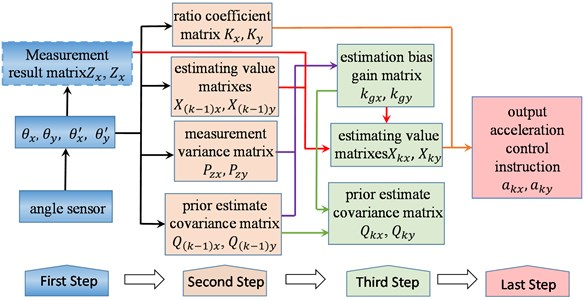Fig. 3Main structure of experimental system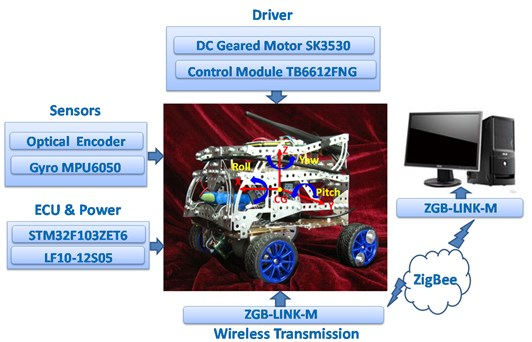A microcontroller (STM32F103ZET6) from STM microelectronics is used for real-time data acquisition and control of the scaled vehicle speed and steering. The microcontroller is installed on the test vehicle. It samples the data from sensors and wirelessly sends them to a ZigBee receiver connected to a computer. MPU6050 from STMicroelectronics, which combines a 3-axis gyroscope, 3-axis accelerometer and a Digital Motion ProcessorTM (DMP), are used to measure acceleration and angle rate, respectively. It is placed near the C.G. of the vehicle, to measure the yaw, roll, pitch angle and rate. The front wheel of vehicle is driven by DC geared motor (SK3530). Microcontroller send control signal to drive motor control module (TB6612FNG). The speed of front wheel (which include right and left side) is measured by E6A2-CS3C speed encoder from OMRON. The photographs of the microcontroller and the sensors are shown in Fig. 3.

Fig. 4Scaled test path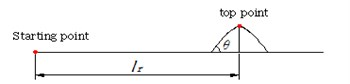a) First experiment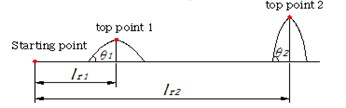b) Second experiment

For the purpose of verifying and revising control model, we programmed the microcontroller to set a series of fixed speed and steering inputs. Thus, identical experiment can be repeated many times. For the first experiment, one obstacle is put ${l}_{r}=$ 2.5 m in the front of starting point, the route of this experiment is show in Fig. 4. The pitch angle of obstacle is $\theta =$22.5°. In this case, vehicle comes close to tip-over. The scaled vehicle differential speed curve, which is controlled by active safety control algorithm (ASC), is shown in Fig. 5.

Fig. 5Scaled vehicle differential speed curve and 2-axis angle with ASC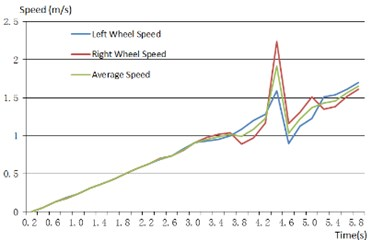a) The speed of each wheel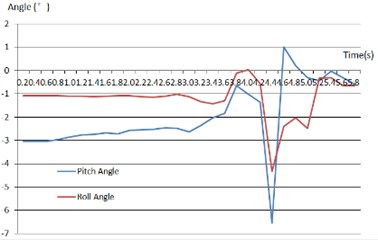b) Pitch and roll angle of vehicle

From above results, when scaled vehicle cross obstacle, ASC make an important effort to adjust the differential speed between right and left wheel, which can verify scaled vehicle body to be stability.

For the second experiment, we put two obstacles on the front route of the vehicle, the scaled vehicle follow path as shown in Fig. 3(b). The first pitch angle is about ${\theta }_{1}=$13.5° and ${l}_{r1}=$1.5 m distance from starting point. The second one is about ${\theta }_{2}=$23.5° and ${l}_{r2}=$2.8 m distance from starting point. The contrast experimental results of ASC and non-control (NC) are shown in following picture.

Fig. 6 is shown variation of pitch angle and average speed when scaled vehicle cross obstacles. If the vehicle was not controlled by active safety control algorithm, at first obstacle it can cross without any violent fluctuate of speed and pitch angle. But it fails to cross the second obstacle and tip-over in the end, which can be implied by pitch angle results. On the contrary, ASC control acceleration of scaled vehicle, pitch angle is adjusted and cross two obstacles successfully.

Fig. 6Contrast curve of Non-control (NC) and Active Safety Control (ASC)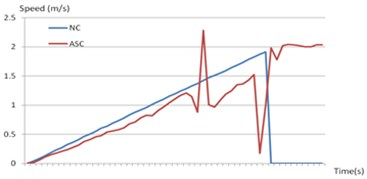a) Pitch angle contrast curve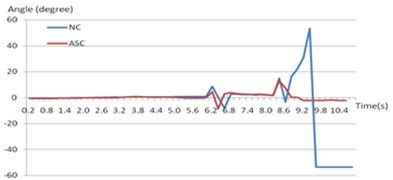b) Average speed contrast curve

## 5. Conclusions

In this paper, a 5-DOF dynamic rollover and tip-over model of orchard vehicle is established as theoretical model working for control method. After that, using Kalman prediction method, the active safety control algorithm is structured, which control 2-axis can accelerate and body posture of vehicle to prevent rollover and tip-over accident. Finally, scaled experimental system was set up, and scaled test prove feasibility and operability of algorithm.

30 October 2016
Accepted
31 October 2016
Published
08 December 2016
SUBJECTS
Vibration in transportation engineering
Keywords
orchard vehicle
rollover
tip-over
active safety control
scaled test
Acknowledgements

Program for The National Natural Science Fund (Grant No. 51405492), Beijing Municipal Science and Technology Commission Project (Grant No. D151100003715001) and Chang Jiang Scholars and Innovative Research Team in University of China (Grant No. IRT13039).